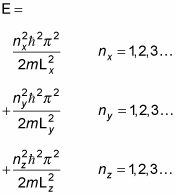##### Quantum Physics For Dummies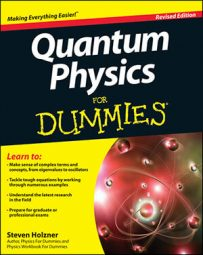In your quantum physics course, you may be asked to normalize the wave function in a box potential. Here's an example: consider the wave functionIn the x dimension, you have this for the wave equation: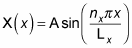So the wave function is a sine wave, going to zero at x = 0 and x = Lz. You can also insist that the wave function be normalized, like this: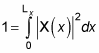By normalizing the wave function, you can solve for the unknown constant A. Substituting for X(x) in the equation gives you the following: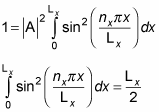Therefore,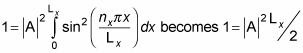which means you can solve for A: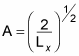Great, now you have the constant A, so you can get X(x):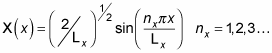Now get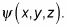You can divide the wave function into three parts: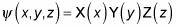By analogy with X(x), you can find Y(y) and Z(z):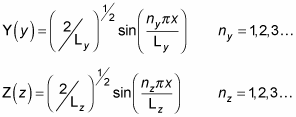So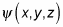equals the following: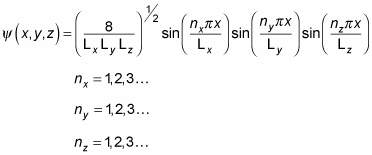That's a pretty long wave function. In fact, when you're dealing with a box potential, the energy looks like this: